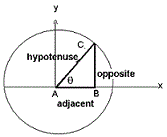### Trigonometry Functions Calculation

Calculate the values for six possible trigonometric functions or ratios as sine, cosine, tangent, cotangent, secant and cosecant against selection, using following formulas:Sinθ = 1 / Cosecθ
Cosθ = 1 / Secθ
Tanθ = Sinθ / Cosθ
Cosecθ = 1 / Sinθ
Secθ = 1 / Cosθ
Cotθ = 1 / Tanθ
 Trigonometry Value: Please Select sin & cos tan & cossin & tancosec & tancot & sincot & coscot & secsincosseccosectancotsec & tancot & cosec
 Sin(x): Cos(x): Tan(x): cosec(x): Sec(x): Cot(x):

The trigonometric functions are functions of an angle and side measurement. It is relate the angles of a triangle to the lengths of its sides.
The summarized table for trigonometric functions and important Formula as follows:
 Function Formula Identities sin(q) Opposite/Hypotenuse 1/cosecθ cos(q) Adjacent/Hypotenuse 1/secθ tan(q) Opposite/Adjacent Sinθ/cosθ cot(q) Adjacent/Opposite 1/tanθ sec(q) Hypotenuse/Adjacent 1/cosθ cosec(q) Opposite/Hypotenuse 1/sinθ